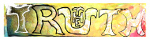发现 ms@ms.lt +370 607 27 665 My work is in the Public Domain for all to share freely. Software Submitted for Space And Time: An Interdisciplinary Approach, Vilnius, September 26-28, 2019. Cognitive Investigation of the Mathematics Which Links Multiple Dimensions Thesis Physical notions of time and space are based on discrete sets of continuous dimensions. Analysis, the mathematics of the continuous, and algebra, the mathematics of the discrete, are related by Lie groups and Lie algebras, respectively. Lie groups are systems of actions, such as rotations, which range over continuous spectrums. Lie algebras and their highly symmetric root systems are the "multiplication tables" which link together such rotations in two, three and higher dimensions. The possibilities are highly constrained and very significant for physics. In particular, there are four infinite families known as the four classical Lie groups and Lie algebras ({$A_n$}, {$B_n$}, {$C_n$}, {$D_n$}). Lie theory is beautiful but, as of yet, offers no intuitive grounds for why there are these four families. A study of the distinctions amongst these four families, by reverse engineering, may yield insights into the cognitive foundations of mathematics, including space and time. Discussion The Lie groups can be thought of as rotations in spaces whose dimensions are described by one-dimensional real numbers ({$B_n$}, {$D_n$}), two-dimensional complex numbers ({$A_n$}), or four-dimensional quaternions ({$C_n$}). Why is the simplest family, {$A_n$}, described by two-dimensional numbers? A combinatorial interpretation of the root systems suggests that the family {$A_n$} models a signal propagating ever forward: {$A_n$}: {$1 < 2 < \dots < n <\dots$} Whereas the other families extend the signal backwards in three possible ways: {$B_n$}: {$\dots -2 < -1 < 0 < 1 < 2 <\dots$} {$C_n$}: {$\dots -2 < -1 < 1 < 2 <\dots$} {$D_n$}: {$\dots -2 < -1 = 1 < 2 <\dots$} This suggests that {$A_n$} models the implicit duality of counting which inherently proceeds both forwards and backwards. These two directions can be explicitly related as if by cutting and, in the case of {$B_n$}, gluing to an external zero, or in the case of {$D_n$}, fusing an internal zero. In the case of {$C_n$}, the duality is doubled as if by folding. The four root systems can also be identified with four ways of interpreting choices. For example, {$A_n$} models choices of the form "choose" vs. "do not choose". Such choices are syntactically asymmetric because "always choose" and "never choose" are syntactically different. Whereas {$D_n$} models choices of the form "choose this" vs. "choose that". Such choices are syntactically symmetric in that "always choose this" and "always choose that" have the same syntactic form, though different semantic content. {$B_n$} and {$C_n$} model intermediate interpretations. These distinctions are relevant for quantum mechanics, where an experimental observer may "not observe", whereas for the observed, there are no such gaps in nature. Conclusion The upshot is that {$A_n$}, {$B_n$}, {$C_n$}, {$D_n$} represent four different ways that the mind can intepret a mathematical expansion as a sequence of choices. They express the symmetries inherent in mathematical expressions themselves. Naujausi pakeitimai Introduction E9F5FC Understandable FFFFFF Questions FFFFC0 Notes EEEEEE 维基百科 影片 书 读物
 Puslapis paskutinį kartą pakeistas 2019 gegužės 16 d., 13:18